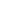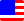Documents  >  Tutorial  >  Literals
Literals

It is a constant written directly in the code.

Constant

int literal

long literal

real literal

string literal

bool literal

Constant

Literals are constants that are written directly in the code.

int i = 10;

string s = "literal";

The value is described directly like above, the instance of the class corresponding to the value is allocated in the memory, and its address is returned.

There are five types of literals:

int literal

long literal

real literal

string literal

bool literal

Literals return instances, so members can be called via literals.

int length = "ggggg".Length; // The length of the string.

int literal

An int literal is a positive integer written directly in the code.

The range is from 0 to the maximum of the int class - 2,147,483,647.

There is no negative literal. If you need a negative number, use the unary minus operator for the literal.

An exception is thrown if the int literal exceeds the above range.

The int literal has the following formats:

A numeric value that a first letter is a digit other than zero and followed by zero or more digits.

123

A number that the above is followed by an uppercase I.

123I

A single zero.

0

long literal

An long literal is a positive integer written directly in the code.

The range is from 0 to the maximum of the long class - 9,223,372,036,854,775,807.

There is no negative literal. If you need a negative number, use the unary minus operator for the literal.

An exception is thrown if the long literal exceeds the above range.

The long literal has the following formats:

A numeric value that a first letter is a digit other than zero and followed by zero or more digits and followed by an upper case L.

123L

A numeric value that a first letter is zero and followed by an upper case L.

0L

real literal

An real literal is a positive real number written directly in the code.

The range is roughly from +5.0e−324 to +1.7e308.

There is no negative literal. If you need a negative number, use the unary minus operator for the literal.

The real literal is evaluated as positive infinity if it is bigger than the above maximum value. The real literal evaluated as 0 if it is smaller than the above minimum value.

The real literal has the following formats:

A numeric value that a first letter is a digit other than zero and followed by zero or more digits and followed by dot and followed by one or more digits.

123.005

A numeric value that a first letter is zero and followed by dot and followed by one or more digits.

0.005

A numeric value that a first letter is dot and followed by one or more digits.

.005

Zero in the real literal is following:

0.0

string literal

A string literal is a string that repeats characters other than double quotes that surrounded by double quotes.

"Other than double quotes"

bool literal

A bool literal is a boolean value written directly in a code. The keyword true and false are used in order to represent bool literals.

true

false